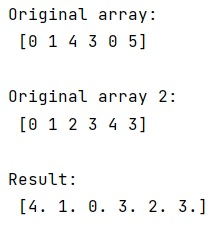# How to sum an array by number in NumPy?

Learn, how to sum an array by number in Python NumPy? By Pranit Sharma Last updated : April 06, 2023

Let us assume we have a numpy array like: [1,2,3,4,5,6] and another array: [0,0,1,2,2,1] and we want to sum the items in the first array by group (the second array) and obtain n-groups results in group number order.

## Summing an array by number in NumPy

For summing an array by number in NumPy, we can use numpy.bincount() which does exactly what we want. This function is used to count the number of occurrences of each value in an array of non-negative ints. The number of bins (of size 1) is one larger than the largest value in the array.

Suppose that we have two arrays, we can pass one array as the input array in bincount() and another as weights as a parameter in bincount.

Let us understand with the help of an example,

## Python code for summing an array by number in NumPy

```# Import numpy
import numpy as np

# Creating an array
arr = np.array([0, 1, 4, 3 ,0, 5])

# Display original array
print("Original array:\n",arr,"\n")

# Creating another array
arr2 = np.array([0, 1, 2, 3, 4, 3])

# Display array 2
print("Original array 2:\n",arr2,"\n")

# Grouping array values and summing the count
res = np.bincount(arr,weights=arr2)

# Display result
print("Result:\n",res,"\n")
```

### Output